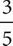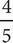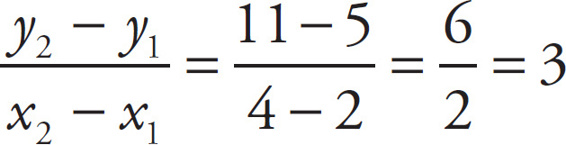# SAT Math Multiple Choice Question 43: Answer and Explanation

### Test Information

Question: 43

3. The graph of line l in the xy-plane passes through the points (2, 5) and (4, 11). The graph of line m has a slope of -2 and an x-intercept of 2. If point (x, y) is the point of intersection of lines l and m, what is the value of y ?

• A.• B.• C. 1
• D. 2

D First, find the slope of line l by using the slope formula:. Plug this slope and one of the points on line l into the slope-intercept form y = mx + b to solve for b, giving you the full equation of the line. If you use the point (2, 5), you get 5 = 3(2) + b or 5 = 6 + b, so b = -1. Therefore, the equation for line l is y = 3x - 1. For line m, the slope is given as -2, and the x-intercept is 2. Be very careful not to jump to the conclusion that equation of line m is y = -2x + 2! In the form y = mx + b, the b is the y-intercept, not the x-intercept. The x-intercept is where y = 0, so you know that (2, 0) is a point on line m. Use this point and the slope to find the equation of line m in the same way you did for line l. 0 = -2(2) + b, so b = 4 and the equation is y = -2x + 4. Now set the x parts of the equations equal to find the point of intersection. If 3x - 1 = -2x + 4, then 5x = 5 and x = 1. Again, be careful! The question asked for the value of y ! Plug x = 1 into one of the line equations to find y. For line l, the equation becomes y = 3(1) - 1 = 3 - 1 = 2, which is (D).